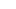# Fibonacci numbers

December 8, 2016

In the Rennaisance, a man called Fibonacci, invented a sucession of numbers: 0, 1, 1, 2, 3, 5, 8, 13, 21, 34, 55… This sucession is consecuence of a number plus his before number. But there more. We can find this number in the nature, for example, in the flowers, because if we count the number of petals in a flower, it should be a fibonacci number. Other example is that if you size the long of the sheet is equal than the fibonacci number multiply from the width of the same sheet

Other example is the conch of the snail, called fibonacci spiral, use the fibonacci sequence.

In the renaissance cathedrals, if you divide the high and the width is fibonacci number

-Starting at 1 digit and "ending" at infinity, each digit value is shared by 4, 5 or 6 numbers in the series. Being 6 only in the case of 1 digit.

-In position elements n, n10, n100, ..., the number of digits increases in the same order. Giving multiple multiples for each number.

Tags:

###### Recent PostsNow is the moment, now is the time

June 2, 2019

1/1